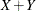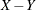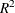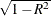### Comments on Interpreting Regression Statistics

In most applications, regression models are merely useful approximations. Reality is often so complicated that you cannot know what the true model is. You might have to choose a model more on the basis of what variables can be measured and what kinds of models can be estimated than on a rigorous theory that explains how the universe really works. However, even in cases where theory is lacking, a regression model can be an excellent predictor of the response if the model is carefully formulated from a large sample. The interpretation of statistics such as parameter estimates might nevertheless be highly problematic.

Statisticians usually use the word prediction in a technical sense. Prediction in this sense does not refer to predicting the future (statisticians call that forecasting) but rather to guessing the response from the values of the regressors in an observation taken under the same circumstances as the sample from which the regression equation was estimated. If you developed a regression model for predicting consumer preferences in 1977, it might not give very good predictions in 2007 no matter how well it did in 1977. If it is the future you want to predict, your model must include whatever relevant factors might change over time. If the process you are studying does in fact change over time, you must take observations at several, perhaps many, different times. Analysis of such data is the province of SAS/STAT procedures such as MIXED and GLIMMIX and SAS/ETS procedures such as AUTOREG and STATESPACE. See Chapter 41: The GLIMMIX Procedure, and Chapter 59: The MIXED Procedure, for more information about modeling serial correlation in longitudinal, repeated measures, or time series data with SAS/STAT mixed modeling procedures. See the SAS/ETS User's Guide for more information about the AUTOREG and STATESPACE procedures.

The comments in the rest of this section are directed toward linear least squares regression. For more detailed discussions of the interpretation of regression statistics, see Darlington (1968); Mosteller and Tukey (1977); Weisberg (1985); Younger (1979).

#### Interpreting Parameter Estimates from a Controlled Experiment

Parameter estimates are easiest to interpret in a controlled experiment in which the regressors are manipulated independently of each other. In a well-designed experiment, such as a randomized factorial design with replications in each cell, you can use lack-of-fit tests and estimates of the standard error of prediction to determine whether the model describes the experimental process with adequate precision. If so, a regression coefficient estimates the amount by which the mean response changes when the regressor is changed by one unit while all the other regressors are unchanged. However, if the model involves interactions or polynomial terms, it might not be possible to interpret individual regression coefficients. For example, if the equation includes both linear and quadratic terms for a given variable, you cannot physically change the value of the linear term without also changing the value of the quadratic term. Sometimes it might be possible to recode the regressors, such as by using orthogonal polynomials, to simplify the interpretation.

If the nonstatistical aspects of the experiment are also treated with sufficient care (such as the use of placebos and double blinds), then you can state conclusions in causal terms; that is, this change in a regressor causes that change in the response. Causality can never be inferred from statistical results alone or from an observational study.

If the model you fit is not the true model, then the parameter estimates can depend strongly on the particular values of the regressors used in the experiment. For example, if the response is actually a quadratic function of a regressor but you fit a linear function, the estimated slope can be a large negative value if you use only small values of the regressor, a large positive value if you use only large values of the regressor, or near zero if you use both large and small regressor values. When you report the results of an experiment, it is important to include the values of the regressors. It is also important to avoid extrapolating the regression equation outside the range of regressors in the sample.

#### Interpreting Parameter Estimates from an Observational Study

In an observational study, parameter estimates can be interpreted as the expected difference in response of two observations that differ by one unit on the regressor in question and that have the same values for all other regressors. You cannot make inferences about changes in an observational study since you have not actually changed anything. It might not be possible even in principle to change one regressor independently of all the others. Neither can you draw conclusions about causality without experimental manipulation.

If you conduct an observational study and you do not know the true form of the model, interpretation of parameter estimates becomes even more convoluted. A coefficient must then be interpreted as an average over the sampled population of expected differences in response of observations that differ by one unit on only one regressor. The considerations that are discussed under controlled experiments for which the true model is not known also apply.

#### Comparing Parameter Estimates

Two coefficients in the same model can be directly compared only if the regressors are measured in the same units. You can make any coefficient large or small just by changing the units. If you convert a regressor from feet to miles, the parameter estimate is multiplied by 5280.

Sometimes standardized regression coefficients are used to compare the effects of regressors measured in different units. Standardized estimates are defined as the estimates that result when all variables are standardized to a mean of 0 and a variance of 1. Standardized estimates are computed by multiplying the original estimates by the sample standard deviation of the regressor variable and dividing by the sample standard deviation of the dependent variable.

Standardizing the variables effectively makes the standard deviation the unit of measurement. This makes sense only if the standard deviation is a meaningful quantity, which usually is the case only if the observations are sampled from a well-defined population. In a controlled experiment, the standard deviation of a regressor depends on the values of the regressor selected by the experimenter. Thus, you can make a standardized regression coefficient large by using a large range of values for the regressor.

In some applications you might be able to compare regression coefficients in terms of the practical range of variation of a regressor. Suppose that each independent variable in an industrial process can be set to values only within a certain range. You can rescale the variables so that the smallest possible value is zero and the largest possible value is one. Then the unit of measurement for each regressor is the maximum possible range of the regressor, and the parameter estimates are comparable in that sense. Another possibility is to scale the regressors in terms of the cost of setting a regressor to a particular value, so comparisons can be made in monetary terms.

#### Correlated Regressors

In an experiment, you can often select values for the regressors such that the regressors are orthogonal (not correlated with each other). Orthogonal designs have enormous advantages in interpretation. With orthogonal regressors, the parameter estimate for a given regressor does not depend on which other regressors are included in the model, although other statistics such as standard errors and p-values might change.

If the regressors are correlated, it becomes difficult to disentangle the effects of one regressor from another, and the parameter estimates can be highly dependent on which regressors are used in the model. Two correlated regressors might be nonsignificant when tested separately but highly significant when considered together. If two regressors have a correlation of 1.0, it is impossible to separate their effects.

It might be possible to recode correlated regressors to make interpretation easier. For example, if X and Y are highly correlated, they could be replaced in a linear regression byandwithout changing the fit of the model or statistics for other regressors.

#### Errors in the Regressors

If there is error in the measurements of the regressors, the parameter estimates must be interpreted with respect to the measured values of the regressors, not the true values. A regressor might be statistically nonsignificant when measured with error even though it would have been highly significant if measured accurately.

#### Probability Values (p-Values)

Probability values (p-values) do not necessarily measure the importance of a regressor. An important regressor can have a large (nonsignificant) p-value if the sample is small, if the regressor is measured over a narrow range, if there are large measurement errors, or if another closely related regressor is included in the equation. An unimportant regressor can have a very small p-value in a large sample. Computing a confidence interval for a parameter estimate gives you more useful information than just looking at the p-value, but confidence intervals do not solve problems of measurement errors in the regressors or highly correlated regressors.

#### InterpretingR square is usually defined as the proportion of variance of the response that is predictable from (can be explained by) the regressor variables. It might be easier to interpret, which is approximately the factor by which the standard error of prediction is reduced by the introduction of the regressor variables.

R square is easiest to interpret when the observations, including the values of both the regressors and response, are randomly sampled from a well-defined population. Nonrandom sampling can greatly distort R square. For example, excessively large values of R square can be obtained by omitting from the sample observations with regressor values near the mean.

In a controlled experiment, R square depends on the values chosen for the regressors. A wide range of regressor values generally yields a larger R square than a narrow range. In comparing the results of two experiments on the same variables but with different ranges for the regressors, you should look at the standard error of prediction (root mean square error) rather than R square.

Whether a given R square value is considered to be large or small depends on the context of the particular study. A social scientist might consider an R square of 0.30 to be large, while a physicist might consider 0.98 to be small.

You can always get an R square arbitrarily close to 1.0 by including a large number of completely unrelated regressors in the equation. If the number of regressors is close to the sample size, R square is very biased. In such cases, the adjusted R square and related statistics discussed by Darlington (1968) are less misleading.

If you fit many different models and choose the model with the largest R square, all the statistics are biased and the p-values for the parameter estimates are not valid. Caution must be taken with the interpretation of R square for models with no intercept term. As a general rule, no-intercept models should be fit only when theoretical justification exists and the data appear to fit a no-intercept framework. The R square in those cases is measuring something different (see Kvalseth 1985).

#### Incorrect Data Values

All regression statistics can be seriously distorted by a single incorrect data value. A decimal point in the wrong place can completely change the parameter estimates, R square, and other statistics. It is important to check your data for outliers and influential observations. Residual and influence diagnostics are particularly useful in this regard.

When a data point is declared as influential or as outlying as measured by a particular model diagnostic, this does not imply that the case should be excluded from the analysis. The label outlier does not have a negative connotation. It means that a data point is unusual with respect to the model at hand. If your data follow a strong curved trend and you fit a linear regression, then many data points might be labeled as outliers not because they are bad or incorrect data values, but because your model is not appropriate.